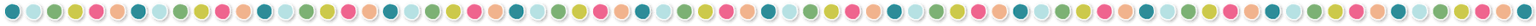# Keep Calm and Use L’Hospital’s RuleIn the last of three posts on Teaching Limits, let’s explore the use of L’Hôpital’s Rule for those who teach Calculus. When Pre-Calculus students use direct substitution that results in 0/0, they know the next step should be to apply one of the algebraic techniques. When Calculus students have studied derivatives, they can explore this new technique as an application of the derivative.

## One word of caution to students:

### Do not confuse L’Hôpital’s Rule with the Quotient Rule.

The Quotient Rule is the first derivative of the entire rational function.  L’Hôpital’s Rule takes the derivative of each part independently of the other.

Spelling Lesson:  I often have teachers ask me to change my notes to spell “Guillaume de l’Hôpital’s” name as “l’Hospital.” So, I did a little research and found this footnote on Wikipedia. “In the 17th and 18th centuries, the name was commonly spelled “l’Hospital”, and he himself spelled his name that way. However, French spellings have been altered: the silent ‘s’ has been removed and replaced with the circumflex over the preceding vowel. The former spelling is still used in English where there is no circumflex.” Not only did I learn about this fact, but I also found it interesting that some believe this rule was really discovered by Johann Bernoulli. Now we have integrated learning for both English and History here.

Be sure to read to the end for a FREE HOT TOPIC Infographic!

## General Procedure for Indeterminate Form:

1. First, know that the limit of a rational function as x approaches c is truly in indeterminate form.
2. If it is, then factor the numerator and denominator.
3. Reduce the expression to simplest form.
4. Use direct substitution again to determine the limit, if it exists.

If you need teaching material for this lesson,  check out my product, L’Hopital’s Rule, here:## FORMAL DEFINITION:

Assume f(x) and g(x) are differentiable on an open interval containing a and that f(c)= g(c)=0 . Also assume that g′(x)≠0 (except possibly at c),  Then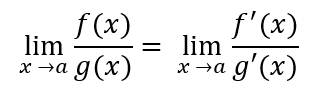If the limit on the right exists or is infinite ( ±∞ ).  This conclusion also holds if f(x) and g(x) are differentiable for x near (but not equal to a)  andAlso, this rule is valid for one-sided limits.

Our problem still stands: If substitution leads to (0/0) or (∞/∞) students must decide whether there is a limit or no limit.  However, now we have a new tool in our tool box.

## General Procedure for applying L’Hôpital’s Rule with Indeterminate Form:

1.  Determine if you can use L’Hôpital’s Rule. There are two conditions to meet. It can only be applied if:

a. You are finding the limit of two functions written in the form of a rational expression f(x)/g(x); and,

b. Direct substitution yields zero divided by zero or infinity divided by infinity.

2. Take the derivative of both the numerator and the denominator.

3. Find the limit of the derivatives, if you get a real number, then the limit exists. This is also the limit of the initial function. However, if you get indeterminate form again,

4. Repeat Steps 2 and 3 again. You can continue to apply the rule repeatedly, as long as the conditions are satisfied.

EXAMPLES: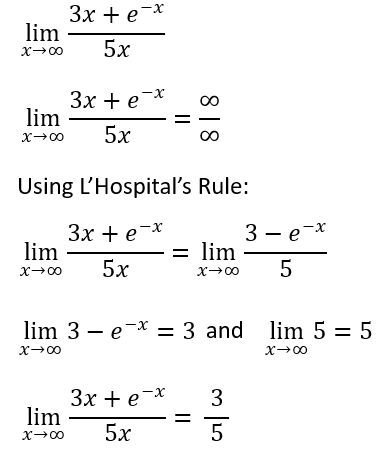## Some things to consider:

1. There are cases where L’Hôpital’s Rule can be applied if you rewrite the limit in proper form, consider these examples: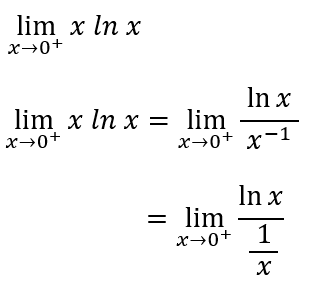L’Hôpital’s Rule can be applied here.  However, if you wrote the limit in the format below, L’Hôpital’s Rule would not simplify the limit.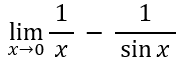2.  Sometimes students will want to apply the rule to every limit that involves rational functions. Explain why the rule cannot be applied!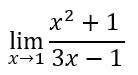3.  There are times when you must apply L’Hôpital’s Rule more than once. Here is such a case:First check that the limit as x approaches zero is in indeterminate form of 0/0.Then, we can apply the rule for a first time.Direct substitution at this point will again return an indeterminate form. So, let’s apply the rule once again and take the second derivative.Once again, using direct substitution, we will arrive at the limit of the function as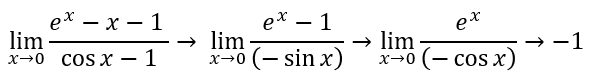4.  Finally, we need our students to have an abstract understanding of this rule. Let’s say you are given some information about a function: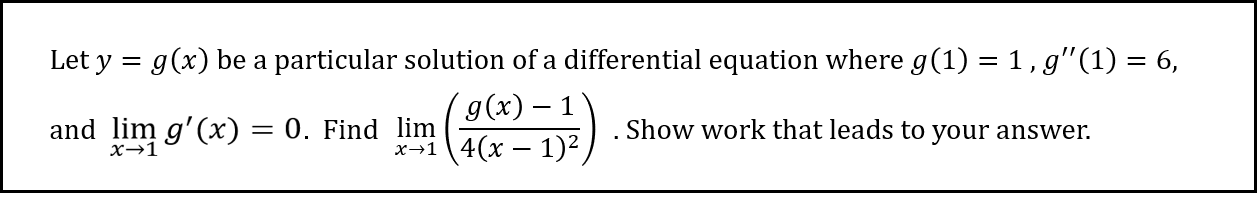First, students need to show that indeterminate form is present.  Namely,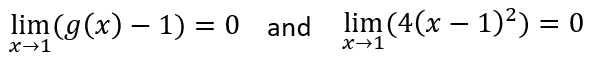Then, the work with proper notation is shown below: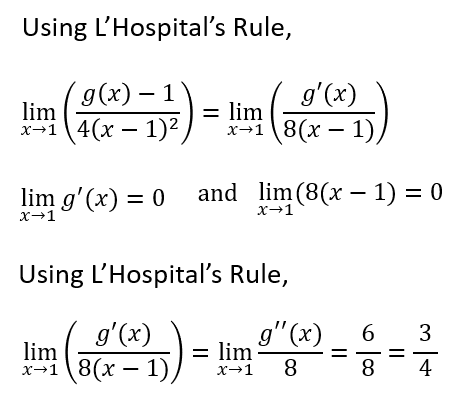Finally, I promised to include another HOT TOPICS FREE INFOGRAPHIC for you and your students! Has this helped you to find a better understanding of how to guide your students? I would love to hear any other tips or suggestions you might have. Please feel free to leave a comment below!

Have you joined our newsletter yet? So much valuable stuff for you and your students! See you next time!## You may also like...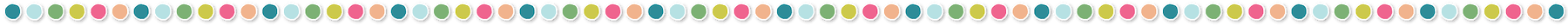This site uses Akismet to reduce spam. Learn how your comment data is processed.

## Let's connect